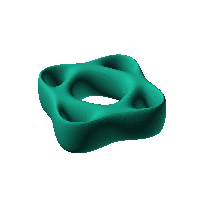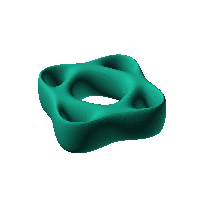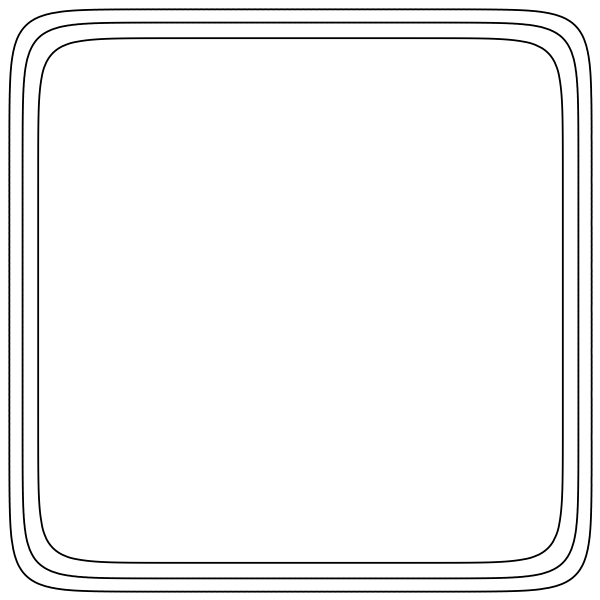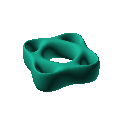Blob Farm# blob19The blob in all its glory:Bretzel surface. It would make a nice case if you filled in the central cavity with something interesting. http : // virtualmathmuseum.org/Surface/bretzel2/bretzel2.html Bretzel5,agenus5tubearoundtwointersectingellipses:f(x,y,z):=((x2+y2/4−1)·(x2/4+y2−1))2+z2/2.

$2\left(\mathrm{x2}+\frac{\mathrm{y2}}{4}-1\right)·\left(\frac{\mathrm{x2}}{4}+\mathrm{y2}-1\right)+\frac{\mathrm{z2}}{2}＝0$

 Click on the snapshot to download the blob's stl file.Octave Code:
```1; # Prevent Octave from thinking that this is a function
# though one is defined here
#  This is a splat shaped blob, but symmetrical

function w = f(x2,y2,z2,c,r,e)
x  = (x2-c(1))/r(1);
y  = (y2-c(2))/r(2);
z  = (z2-c(3))/r(3);
# function at origin must be <0, and >0 far enough away.  w=0 defines the surface
w=((x.^2 + (y.^2)/4 - 1).*((x.^2)/4 + y.^2 - 1)).^2 + z.^2/2 - 0.0./(x.^4 + y.^4 + z.^4)-0.15  ;

endfunction;

```
GNU Octave